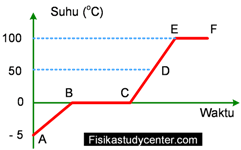# Fisika Study Center

## Black Principle on Heat Transfer

physics.fisikastudycenter.com_2021  Black principle on a block of copper and water to find the final temperature.

Problem 1

500 gram of copper at 33.8°C is heated to a temperature of 100° C, what is the heat taken in this process? Use the specific heat of copper is 385 J/kg °C!

## Converting Temperatures

Sample questions and solutions for temperature converting. These samples are for converting between the kelvin and celcius, celcius and reamur, and fahrenheit thermometer.

Question 1
a) 127 °C = ....K
b) 1000 K =......°C## Application of Lenses : Camera

We know in common the usage of lenses in magnifiers, cameras, microscopes and telescopes. These four optical equipments discussed in most physics textbooks for yunior or high school students and have lenses as the vital parts.Actually there are more and more equiptments using lens or lenses for component for instance eye glasses, contact lens, projector, printer, scanner, periscope. In this short article we will discussed a camera as our topic.

## 6 Common Problems of Material Density

physics.fisikastudycenter.com - Learning 5 Common problems of material density, grade 7 of yunior high school physics.

Density
The density of a material is equal to its mass divided by its volume:where:
ρ = density in kg/m3
m = mass in kg
V = volume in m3

Problem 1
A body of 300 g with a volume of 400 cm3. Find the density of the body show your answer in kg/m3!

## 9 Common Problems of Heat for Junior

physics.fisikastudycenter.com - Learning heat for junior hingh school students. Given 9 common problems includes the solutions, the specific heat, the heat of fusion and black principle in heat exchange will be discussed.Problem 1
Water with a mass of 100 gram at a temperature of 20° C then heated to a temperature of 80° C. If the apecific heat of water is 1 cal/gr ° C what is the heat needed in this process show your answer in calorie!

### More Articles...

Joomla Templates: by JoomlaShack.com